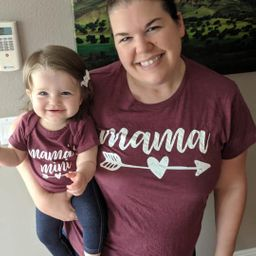Our Discord hit 10K members! 🎉 Meet students and ask top educators your questions.Join Here!Numerade Educator

# Find the mean, median, and mode.$$\begin{array}{l}18,5,8,22,16,8,15,22,12\end{array}$$

## mean $=14,$ median $=15$ and mode $=8,22$

#### Topics

No Related Subtopics

### Discussion

You must be signed in to discuss.

### Video Transcript

let's first start off by finding them even or the average. When we add all of these numbers up, we get 1 26 divided by the number of numbers in our list, which is nine. And that leaves us with 14. Next, we can find the mode. That's the number that occurs the most amount of times we can see. The number eight occurs twice in the number 22 occurs twice, so the mood would be eight and 22. Lastly, to find the media and we have to rearrange this in numerical order, so we'll start with the lowest number and then, well, list them. In least agreed a supporter 16 then 18 then 20 to another 22. The median would be the number that's directly in the middle, which would be 16Other Schools

#### Topics

No Related Subtopics